# Estimating wind speed

#### 2016-10-19

In this vignette we will analyse the example data from the moveWindSpeed package. In order to do so we will first load the data and explore it before diving in to the analysis.

require(moveWindSpeed)
## Loading required package: moveWindSpeed
## Loading required package: move
## Loading required package: geosphere
## Loading required package: sp
## Loading required package: raster
## Loading required package: rgdal
## rgdal: version: 1.1-10, (SVN revision 622)
##  Geospatial Data Abstraction Library extensions to R successfully loaded
##  Loaded GDAL runtime: GDAL 1.11.3, released 2015/09/16
##  Path to GDAL shared files: /usr/share/gdal/1.11
##  Loaded PROJ.4 runtime: Rel. 4.9.2, 08 September 2015, [PJ_VERSION: 492]
##  Path to PROJ.4 shared files: (autodetected)
##  Linking to sp version: 1.2-3
data("storks")
plot(
storks,
xlab = "Longitude",
ylab = "Latitude",
main = "Stork data" ,
pch = 19,
cex = .3
)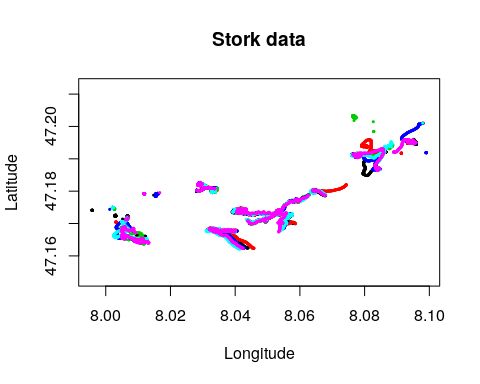## Investigate one individual

We focus on 2 minutes where we know that high resolution trajectories were recorded while the bird was in a thermal and select the first individual. We transform the trajectory to a local projection for better plotting.

storksSub <-
storks[strftime(timestamps(storks), format = "%H%M", tz="UTC") %in% c("1303", "1304"), ]
storksWind <- getWindEstimates(storksSub)
storksWind <- spTransform(storksWind, center = T)
indiv1 <- storksWind[]
indiv1
## class       : Move
## features    : 120
## extent      : -246.2953, 313.526, -55.89234, 45.84754  (xmin, xmax, ymin, ymax)
## coord. ref. : +proj=aeqd +ellps=WGS84 +lon_0=8.03556635 +lat_0=47.16786075
## variables   : 20
## names       : eobs_horizontal_accuracy_estimate, eobs_speed_accuracy_estimate, eobs_status, eobs_temperature, eobs_type_of_fix, eobs_used_time_to_get_fix, ground_speed, heading, height_above_ellipsoid, manually_marked_outlier,  event_id, estimationSuccessful, residualVarAirspeed,    windX,        windY, ...
## min values  :                              1.54,                         0.13,           A,               19,                3,                       179,         3.63,    1.97,                  792.2,                      NA, 384663102,                 TRUE,          0.07235049, 4.899265,  0.019793566, ...
## max values  :                              1.79,                         0.26,           A,               19,                3,                       299,        16.56,  355.86,                  983.7,                      NA, 384663221,                 TRUE,          0.23316526, 6.136631, -0.021843835, ...
## timestamps  : 2014-08-18 15:03:00 ... 2014-08-18 15:04:59 Time difference of 2 mins  (start ... end, duration)
## sensors     : GPS
## indiv. data : individual_id, tag_id, id, comments, death_comments, earliest_date_born, exact_date_of_birth, latest_date_born, local_identifier, ring_id, sex, taxon_canonical_name
## indiv. value: 21977647 21232176 21977700 4 nestlings. AN873 is the youngest. died 2015-01-11 on landfill Dos Hermanas / S-Spain. Weak strange movements for about 6 hrs. Carcass found, no external injuries. Poisoned? lat: 37.2198845; long: -5.8800427
##  NA NA 2014-04-15 00:00:00.000 Bubbel + / DER AN873 (eobs 3666) DER AN873 f NA
## unused rec. : 303
## date created: 2016-10-14 10:38:48

Lets first assure that the individual is sampled with one hertz. This is crucial because some of the calculations we do below for plotting assume this.

unique(as.numeric(diff(timestamps(indiv1)), units='secs'))
##  1

Now lets have a look at the track in one individual within the thermal. We add an arrow to each 10th location to indicate the estimated wind speed.

plot(
indiv1,
type = 'l',
xlim = c(-150, 150),
main = row.names(idData(indiv1))
)
s <- as.numeric(timestamps(indiv1)) %% 10 == 0
expansion <- 3
arrows(
coordinates(indiv1)[s, 1],
coordinates(indiv1)[s, 2],
coordinates(indiv1)[s, 1] + indiv1$windX[s] * expansion, coordinates(indiv1)[s, 2] + indiv1$windY[s] * expansion,
col = 'red',
length = .1
)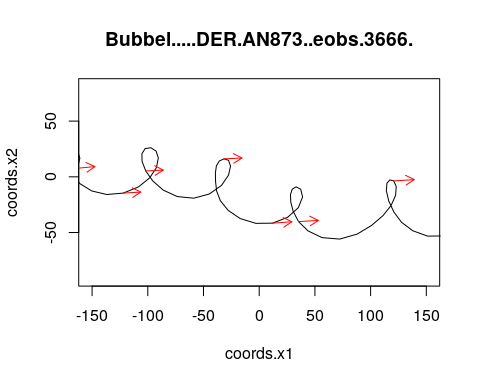We can also select one rotation and plot how head wind speed vector combined with the airspeed vector results in the ground speed.

indivSub <- indiv1[45:72,]
plot(indivSub, pch = 19)
arrows(
coordinates(indivSub)[-n.locs(indivSub), 1],
coordinates(indivSub)[-n.locs(indivSub), 2],
coordinates(indivSub)[-1, 1],
coordinates(indivSub)[-1, 2],
length = .1
)
arrows(
coordinates(indivSub)[-n.locs(indivSub), 1],
coordinates(indivSub)[-n.locs(indivSub), 2],
coordinates(indivSub)[-n.locs(indivSub), 1] + head(indivSub$windX,-1), coordinates(indivSub)[-n.locs(indivSub), 2] + head(indivSub$windY,-1),
col = "red",
length = .1
)
arrows(
coordinates(indivSub)[-n.locs(indivSub), 1] + head(indivSub$windX,-1), coordinates(indivSub)[-n.locs(indivSub), 2] + head(indivSub$windY,-1),
coordinates(indivSub)[-1, 1],
coordinates(indivSub)[-1, 2],
col = "green",
length = .1
)
legend(
"topright",
legend = c("Ground speed", "Wind Speed", "Air Speed"),
bty = "n",
col = c("black", "red", "green"),
lty = 1
)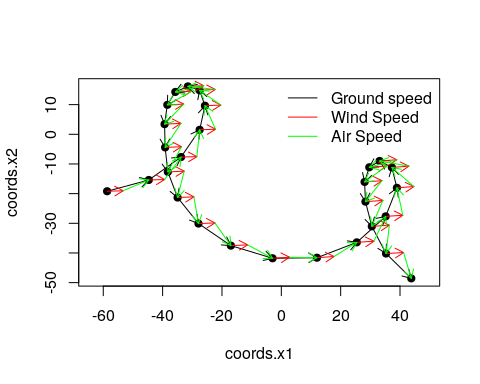Now lets see how the track looks once we subtract the wind speed, so that we only look at the rotations relative to the air. We see that the bird half way the thermal changes its relative position in the air column.

s <- !is.na(indiv1$windX) x <- cumsum(diff(coordinates(indiv1)[, 1])[s] - indiv1$windX[s])
y <- cumsum(diff(coordinates(indiv1)[, 2])[s] - indiv1$windY[s]) plot(x, y, type = 'l', main = "Wind corrected trajectory")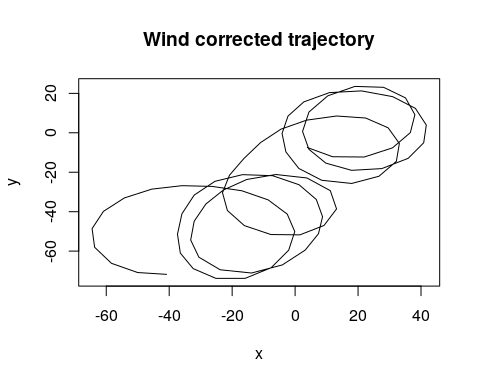## Multiple storks in a thermal Lets take the same two minute section and compare all wind speed estimates. We see that there all estimates fall in a pretty narrow range. storksSub <- getWindEstimates(storksSub, windowSize = 31) plot( storksSub$windX,
storksSub$windY, col = trackId(storksSub), pch = 19, xlim = range(na.omit(c(0, storksSub$windX))),
ylim = range(na.omit(c(0, storksSub$windY))), asp = 1, main = "Distribution of wind speed estimates" )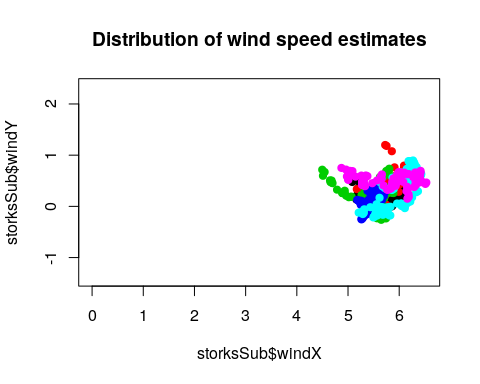We can also create a vertical wind speed profile through a thermal. To do so we select a thermal where most birds are present. We see that there seems to be quite a consistent pattern. windData <- getWindEstimates(storks) windData <- windData[strftime(timestamps(windData), format = "%H", tz="UTC") == "12" & !is.na(windData$windX), ]
plot(
sqrt(windData$windX ^ 2 + windData$windY ^ 2),
windData$height_above_ellipsoid, main = "Vertical wind profile", xlab = "Wind speed", ylab = "Altitude", col = trackId(windData), pch = 19 )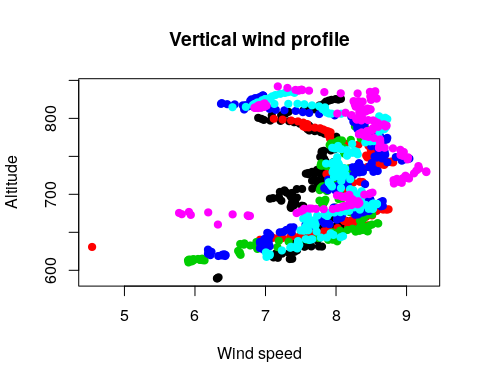## Varying the settings We see that if we raise our criteria for thermal soaring the conditions are more rarely occurring. indivSub <- storks[] quater <- getWindEstimates(indivSub, hasVariationInHeadingFunction = getDefaultIsThermallingFunction(90)) half <- getWindEstimates(indivSub, hasVariationInHeadingFunction = getDefaultIsThermallingFunction(180)) full <- getWindEstimates(indivSub, hasVariationInHeadingFunction = getDefaultIsThermallingFunction(360)) two <- getWindEstimates(indivSub, hasVariationInHeadingFunction = getDefaultIsThermallingFunction(720)) sum(!is.na(quater$windX))
##  2281
sum(!is.na(half$windX)) ##  1635 sum(!is.na(full$windX))
##  706
sum(!is.na(two$windX)) ##  159 We can for example also focus on a longer window, meaning we find are more likely to find hits for our rotation criteria. short <- getWindEstimates(indivSub, hasVariationInHeadingFunction = getDefaultIsThermallingFunction(720), windowSize = 29) long <- getWindEstimates(indivSub, hasVariationInHeadingFunction = getDefaultIsThermallingFunction(720), windowSize = 41) sum(!is.na(short$windX))
##  159
sum(!is.na(long\$windX))
##  321

We can also speed up calculations by only looking at each 5th location. This will also reduce the overlap between windows.

system.time(getWindEstimates(
indivSub,
isFocalPoint = function(i, ts) {
T
}
))
##    user  system elapsed
##   6.288   5.136   4.222
system.time(getWindEstimates(
indivSub,
isFocalPoint = function(i, ts) {
(i %% 5) == 0
}
))
##    user  system elapsed
##   1.220   1.092   0.758